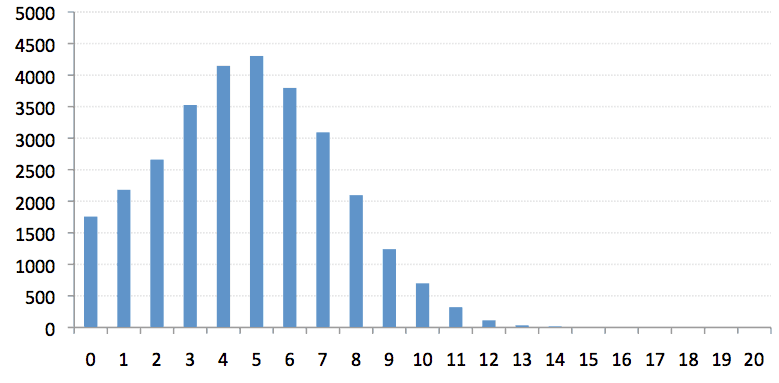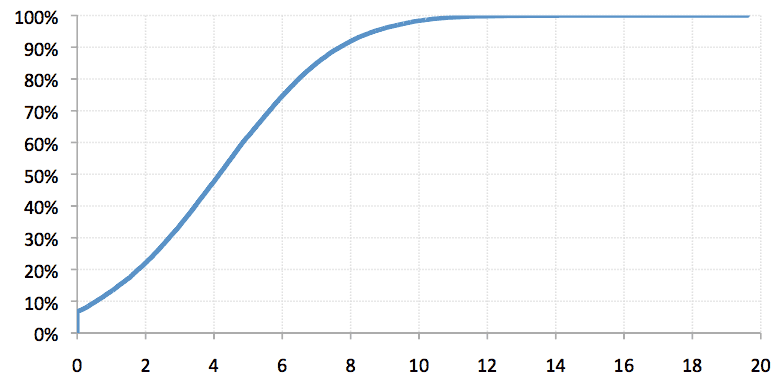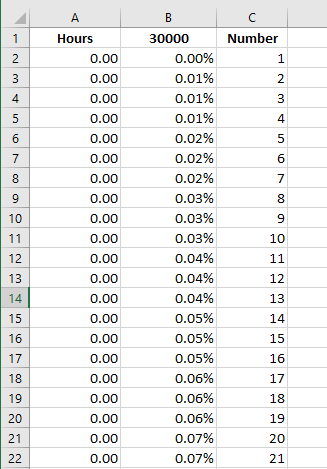### Cumulative Distribution Function Charts in Excel

Most analysts know what a histogram is: it’s a chart where each piece of data is put into one of several bins and then the bin counts are plotted in order to gain insight into how the data is distributed. Histograms are useful as they have an intuitive appeal because it’s easy to see how the data is distributed just by looking at the chart. Of course, they aren’t perfect. The whole binning process is pretty subjective and depending on how the bins are selected the results can be different, which is deceptive. If only there was an alternative that could address these shortcomings…

Well, there is: histograms have an underutilized sibling known as the Cumulative Distribution Function (CDF) chart which compliments and, I think, improves on the histogram by doing away with some of the histogram’s problems and offering some useful innovations of its own. Namely, the ability to read off the chart what percentage of the data falls between two points, something that is not possible with a histogram. In this post I will discuss why I think you should make the CDF part of your analytical toolbox and also demonstrate how easy it is to create a CDF in Excel.

Convince me

First, two example charts that describe my dataset: a completely made up list of 30,000 calls-for-service and how long they last from initial call to the closing off of the incident. The first chart is a standard histogram and the second is the corresponding CDF. For both charts the horizontal axis is the same: it’s hours. For the histogram the vertical axis is a count of how many calls are in each bin while the vertical axis of the CDF is a measure of percentage (why percentage will become clear in a bit).So the histogram is the same old boring story. We have a normal distribution that is skewed to the left (fatter on the left than the right). Looks like most calls take about five hours and only a few go above 12 hours. Yawn.Ok, the CDF looks great. But what’s it good for? What can it tell us that the histogram can’t? Remember that I mentioned you can use a CDF to determine what percentage of the data falls between two points? Let’s explore that now.

Suppose I want to know how many calls take less than 5 hours (that is, between 0 and 5 hours). I can read that right off the graph by going across the horizontal axis until I reach 5 and then going up until I hit the trend line. I then read across to the vertical axis and see that the value is about 60%. What this means is that 60% of all calls take 5 hours or less. That’s a much firmer number than ‘the most calls are around 5 hours’. Similarly, what if the question was how many calls take between 5 and 10 hours. Well, first read off the 10 hour value, which is about 99% and we know the 5 hour value is 60% so just take the difference 99-60 to arrive at 33% of all calls take between 5 and 10 hours. Let’s see you do that with a histogram (hint: you can’t do it).

I’m convinced. How do I make one in Excel?

Creating a CDF is very straightforward and the following five steps describe the entire process. I’ve included a quick Excel screen grab to illustrate what the first few rows of the data will look like when you’re done (it’ll make more sense when you’re done the steps).Step One: Start with your data in Column A in Excel sorted from smallest value to largest. My data had a lot of zero values, so that is why the column starts off with a series of zeros.

Step Two: In Column C (yes I intentionally skipped B) put the number 1 in C1, 2 in C2, 3 in C3, etc.  Here’s a useful hint to speed this up: use =C1+1 for C2 and fill down.

Step Three: In B1 enter how many data points you have. I had 30,000 so I dropped that number in there.

Step Four: For B2 use the formula =C2/\$B\$1 and fill down. The idea is that you want to divide the value in Column C by the total count. When you get to the bottom of your data the last value should be 1.

Step Five: Now that the data has been prepped highlight all of columns A and B and insert a new Scatter chart. What you’ll get is the monotonically increasing (i.e. always going up) trend line. You can edit the styling so that the graph is a line rather than points and make whatever other changes make it look it pretty. I recommend fixing the vertical axis at 1 (since the data can never go above 100%) and the minimum horizontal axis at 0, if that’s appropriate. Voila, now you have a CDF chart.

But how do CDFs address the histogram’s shortcomings? Did you notice any bins during the calculation? Nope. CDFs use the value of every data point and therefore do away with binning and the loss of information binning introduces. And because there are no bins the shape of the CDF can’t change like it can in a histogram so that source of subjectivity is neatly side-stepped. And you want to know what else is great? It’s easy to compare two data sets with CDFs by simply plotting them on the same chart. Comparing two histograms on the same plot is not particularly enlightening besides noting how the peaks compare.

In conclusion, CDFs are like grilled cheese: great and easy. And like grilled cheese everyone should try one at lunch (or whenever you next do a frequency analysis). The CDF is a great compliment to traditional histogram analysis and will hopefully become a valuable tool in your graphic analysis toolbox.

An olive branch to histograms

Making a histogram is actually more work than creating a CDF. But if you really want to create one you can either use Microsoft’s Analysis ToolPak (what happened to the ‘c’ MS?) or perform some more elaborate Excel JuJu. Good luck.